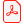# Konferensartikel

## Methods for Linking the Semantic Space and the Space of Properties in Kansei Engineering

Luis Almagro
Technical University of Catalonia, Spain

Irene Subirats
Technical University of Catalonia, Spain

Xavier Tort-Martorell Llabrés
Technical University of Catalonia, Spain

Ladda ner artikelhttp://www.ep.liu.se/ecp_article/index.en.aspx?issue=026;article=089

Linköping Electronic Conference Proceedings 26:89, s.

Visa mer +

ISBN:

ISSN: 1650-3686 (tryckt), 1650-3740 (online)

### Abstract

In the synthesis phase of a kansei engineering study emotions expressed by kansei words are linked to product properties. Two quite different techniques are widely used in this step: quantification theory type I (QT1) and rough sets theory (RS).

QT1 is a kind of linear multiple regression analysis; first proposed by Chikio Hayashi (Hayashi 1952). Categorical variables (properties from our products) are converted into dummy variables. The coefficients of these variables are then calculated. These coefficients are called category scores (CS); and show in which direction one should move an item to get the desired effect on the kansei word. A partial correlation coefficient for each item is calculated; the bigger; the more important it is in the effect on the kansei word studied. Also a multiple correlation coefficient (MCC); equivalent to R2 in linear regression; is calculated; showing the whole validity of the model. In this paper a MATLAB algorithm has been used to calculate category scores; based on a proposal by Yutaka Tanaka (Tanaka 1979).

Rough sets theory (RS) was first introduced by Zdzislaw Pawlak in the early 1980s. Pawlak gives a good review on notions and advances in rough sets theory in a posthumous paper (Pawlak; Skowron 2007). The idea behind RS is using iterative algorithms for extracting rules that classify all the data into groups. In kansei engineering; RS is used to extract which group of product properties evoke a particular kansei. In this paper the software programme ROSE2 has been used to extract the rules using RS. ROSE2 has been implemented by the Laboratory of Intelligent Decision Support Systems of the Poznan University of Technology. (Laboratory of Intelligent Decision Support Systems 2007)

Simon Schütte (Schütte 2004) offers a clear introduction and examples on how to use QT1 and RS in kansei engineering studies.

### Nyckelord

Quantification Theory Type I; Rough Sets Theory; synthesis phase in kansei studies

### Referenser

Inga referenser tillgängliga